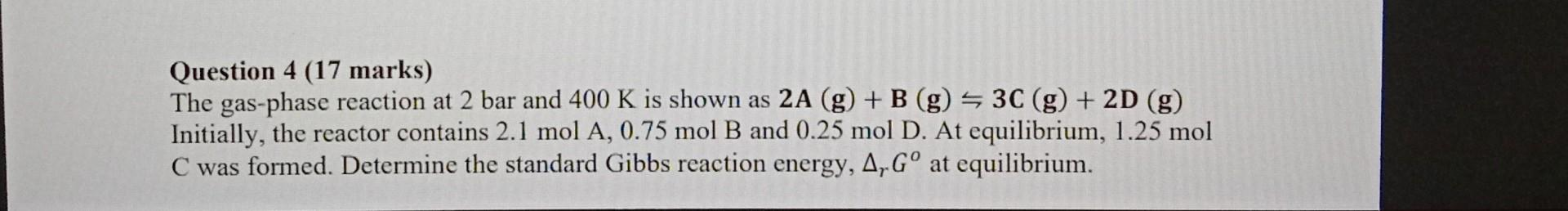Home / Expert Answers / Chemical Engineering / nbsp-question-4-17-marks-the-gas-phase-reaction-at-2-bar-and-400-mathrm-k-is-shown-a-pa365

# (Solved):   Question 4 (17 marks) The gas-phase reaction at 2 bar and $$400 \mathrm{~K}$$ is shown a ...Question 4 (17 marks) The gas-phase reaction at 2 bar and $$400 \mathrm{~K}$$ is shown as $$\mathbf{2 A}(\mathbf{g})+\mathbf{B}(\mathbf{g}) \leftrightharpoons \mathbf{3 C}(\mathbf{g})+\mathbf{2 D}(\mathbf{g})$$ Initially, the reactor contains $$2.1 \mathrm{~mol} \mathrm{~A}, 0.75 \mathrm{~mol} \mathrm{~B}$$ and $$0.25 \mathrm{~mol} \mathrm{D}$$. At equilibrium, $$1.25 \mathrm{~mol}$$ $$\mathrm{C}$$ was formed. Determine the standard Gibbs reaction energy, $$\Delta_{r} G^{0}$$ at equilibrium.

We have an Answer from Expert